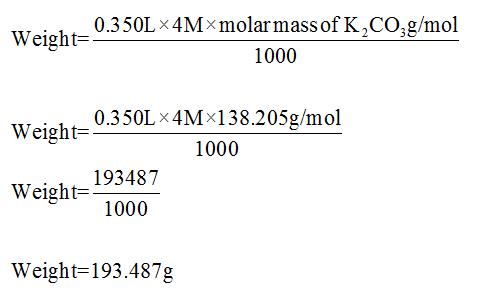# How many grams of K2CO3 would you need to make 350 ml of 4M K2CO3?

Question
63 views

How many grams of K2CO3 would you need to make 350 ml of 4M K2CO3?

check_circle

Step 1

Given data,

Molarity = 4M

Total volume of solution =350mL (0.35L)

It is known that, molar mass of K2CO3=138.205 g/mol

Step 2

The required grams (weight) of K2CO3 can be calculated as...

### Want to see the full answer?

See Solution

#### Want to see this answer and more?

Solutions are written by subject experts who are available 24/7. Questions are typically answered within 1 hour.*

See Solution
*Response times may vary by subject and question.
Tagged in

### Chemistry